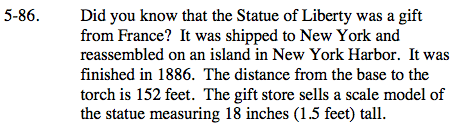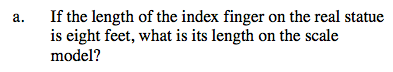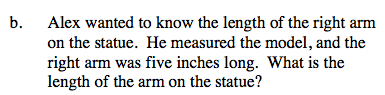### Home > CAAC > Chapter 5 > Lesson 5.2.3 > Problem5-86

5-86.Set up a proportion using the information in the problem.

$\frac{\text{statue}}{\text{model}} = \frac{152}{1.5} = \frac{8}{x}$

Now solve for x.

x = 0.08 ft or about 1 inchFollow the steps in part (a).